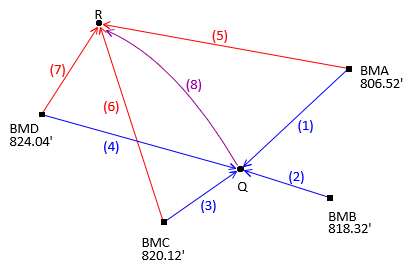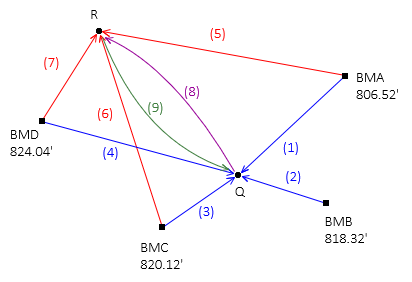## 3. Will Brute Force Work in All Situations?

Add an observation, dElev(8)=-12.47, between points Q and R, Figure B-2.Figure B-2 Observation Added

A fourth elevation for point R must be computed from point Q using the eighth observation. But which elevation of point Q should be used? Any of them? All of them? Its average?

The issue is more fundamental. When two unknowns are connected with a measurement, both are affected; after all, it's a network. Even though the observation is from point Q to point R, point Q's elevation is also affected. So point R's elevation depends on point Q's and point Q's elevation depends on point R's....

Adding another observation in the opposite direction from point R to point Q, Figure B-3, further complicates it.Figure B-3Another Observation Addition